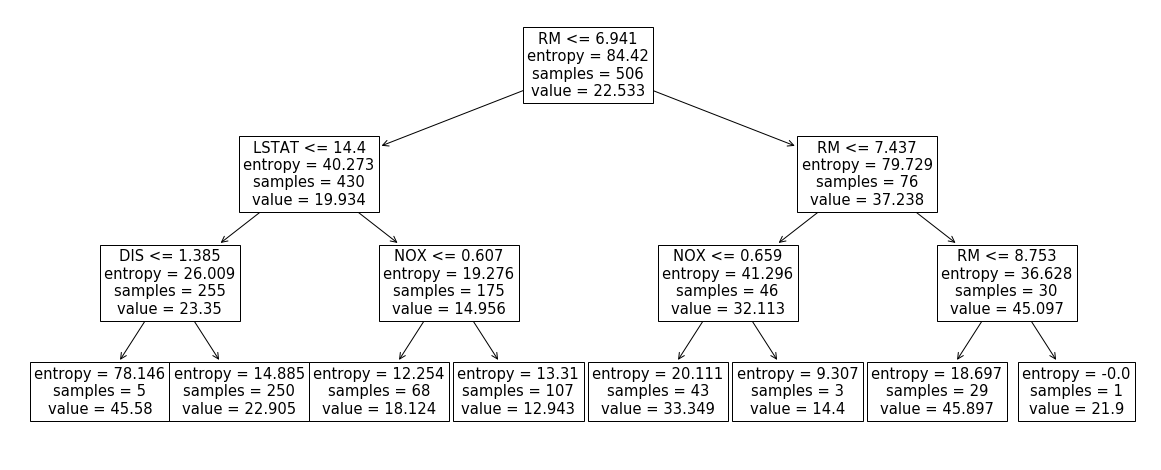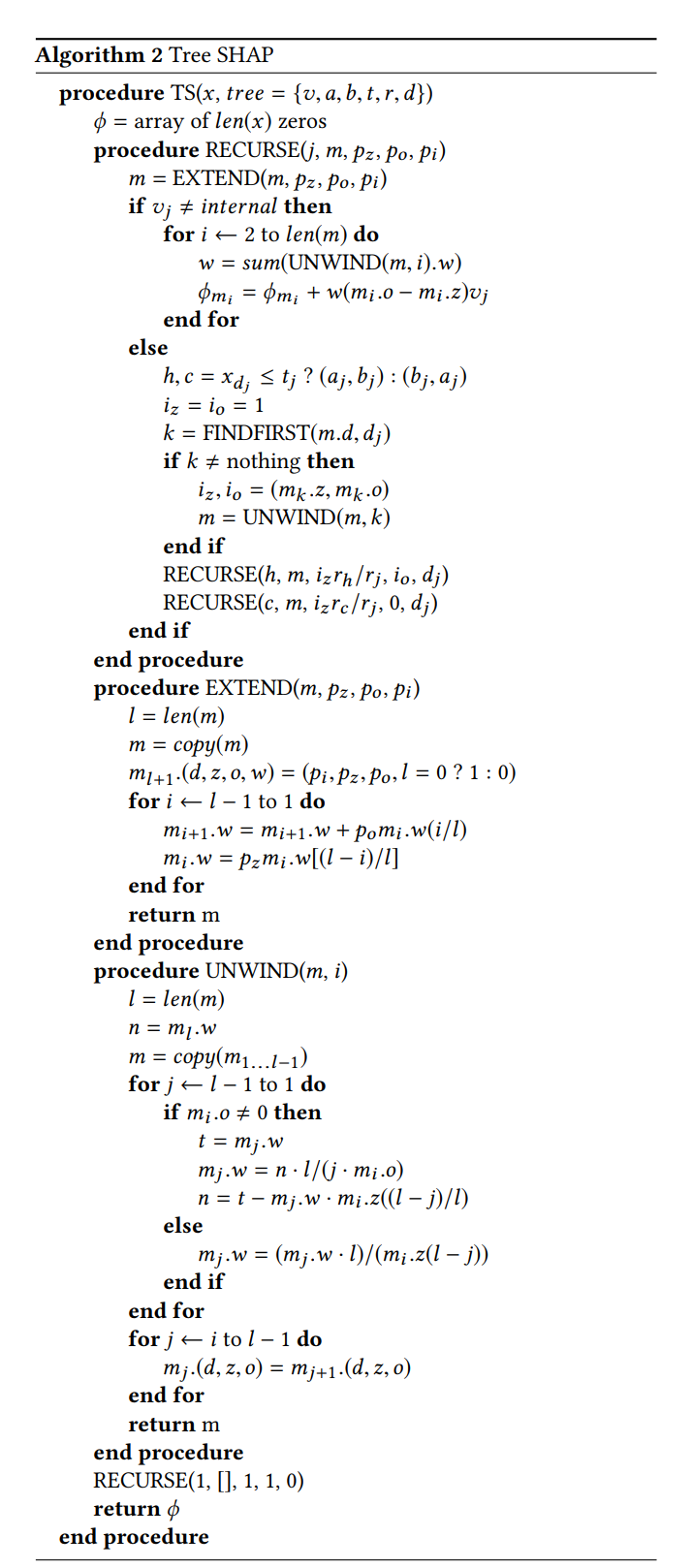## Computing SHAP values from scratch

Recently I tested shap feature importances. SHAP is a novel way of computing which features contributed the most towards the prediction. Whereas the method itself can be generalised to any classifier, it is especially well-suited for tree-based models.

In this blog post I will try to explain what SHAP values are and why I find them very compelling. Following Feynman’s “what I cannot create, I do not understand”, let’s try to compute SHAP feature contributions via a simple example.

Above I grabbed a Boston dataset, restricted it to 4 columns ['RM', 'LSTAT', 'DIS', 'NOX'] and built a decision tree, depicted below.First, let’s compute the shap values for the first row using the official implementation:

shap_value feature_value
RM -2.3953 6.575
LSTAT 2.46131 4.98
DIS -0.329802 4.09
NOX 0.636187 0.538

Note: one nice property of SHAP contributions is that their sum + sample mean is the same as the prediction:

Below we’ll figure out why that’s the case. For now, let’s start on computing those values “by hand”.

# Shapley values

The underlying computation behind shap feature contributions is powered by a solution taken from game theory. The setup in our case is as follows: $$N=4$$ features are now allowed to form coalitions. The worth, $$v$$, of a coalition ['RM', 'LSTAT'] is simply

$v(RM, LSTAT) = E[y|RM=6.575,LSTAT=4.980]$

i.e. the prediction of the classifier if only values of RM and LSTAT are known. Following

$\varphi _{i}(v)={\frac {1}{N}}\sum _{S\subseteq N\setminus \{i\}}{\binom {N-1}{|S|}}^{-1}(v(S\cup \{i\})-v(S))$

solution from the wikipedia page we can now redistribute total “winnings”, $$v(RM, LSTAT, DIS, NOX) - v()$$, fairly so that each feature $$i$$ gets $$\varphi_{i}$$ contribution. Remark that total “winnings” is simply $$E[y|LSTAT=4.98,NOX=0.538,DIS=4.09,RM=6.575] - E[y]$$

where

and

In English, we want to attribute how much we deviate from the naive prediction (sample mean) to each feature. Expanding the formula above we get that feature contribution of RM, $$4 \varphi_{i}$$, is

For the sake of brevity, I would like to omit the explanation of the intuition behind such formula. However I found skim-reading this article and watching this video helping me with understanding why Shapley values are calculated this way.

## Figuring out conditional expectations

The remaining question is now how can we compute the conditional expectations in the above formula. Remember I said that SHAP method can in principle be applied to any classifier? The reason behind this is that

is just a prediction if DIS value for the feature was not known. We could, in theory, retrain without the DIS feature and get a classifier to output this quantity. Or, alternatively, we could marginalise by ranging over observed DIS values, getting a prediction, and taking a weighted sum according to how common the value of DIS is. However, all of those methods are prohibitively slow. In the past I tried to approximate this quantity with the mode of DIS distribution: however it only worked in a binary classification case.

Turns out in a tree-based classifier, such quantity is easy to compute. Look at our tree again:Our row forces us to walk the leftmost path of the tree. However, once we hit the DIS <= 1.385 node we’re not sure where to go as DIS is unknown. Solution: go both left and right and take a weighted sum. In other words:

Let’s write some code that does this for us:

## Putting it all together

shap_value my_shap feature_value
RM -2.3953 -2.3953 6.575
LSTAT 2.46131 2.46131 4.98
DIS -0.329802 -0.329802 4.09
NOX 0.636187 0.636187 0.538

And there you have it: we have replicated the computations in the shap library. When SHAP values are computed for a forest of decision trees, we simply average those. Because SHAP contributions are Shapley values we get certain desired properties “for free”:

• contributions + bias = prediction
• contributions of irrelevant features are 0
• consistency: changing a model so a feature has a larger impact on the model will never decrease the contribution assigned to that feature

and thus I have become an advocate for using this method.

# Final thoughts

In my eyes, the genius of Scott M. Lundberg, Gabriel G. Erion, and Su-In Lee who published the shap library isn’t that they used Shapley values: this has been known prior to them by Strumbelj, Erik, and Igor Kononenko all the way back in 2014, but the fact that they invented a fast pred_tree algorithm. The primitive recursive algorithm presented in this blog post would be too slow to deploy in a live system for any reasonably-sized tree. When faced with a similar problem of computing conditional expectations in the past and I ended up approximating these by going down the path that is closest to the current value instead of taking the weighted average of the children. My hacky solution makes computations of contributions just as fast as predictions however it is less robust. I include the fast “Algorithm 2” from the tree shap paper, but, after staring at it for a few days by now, I have given up on any hope of understanding it just by reading it. Perhaps some motivated reader could email me the explanation. For now, this is good bye!